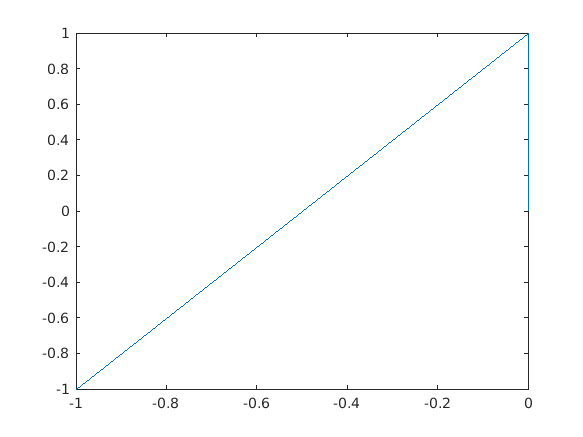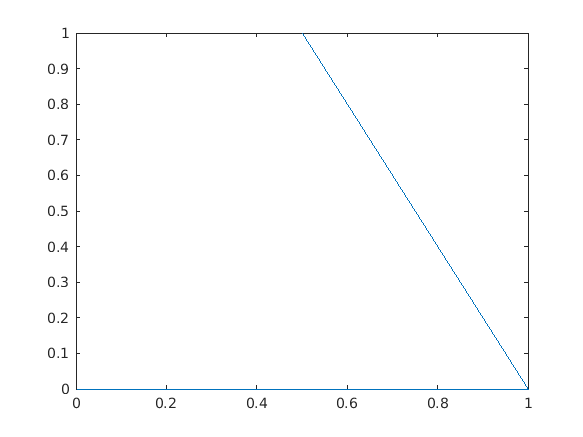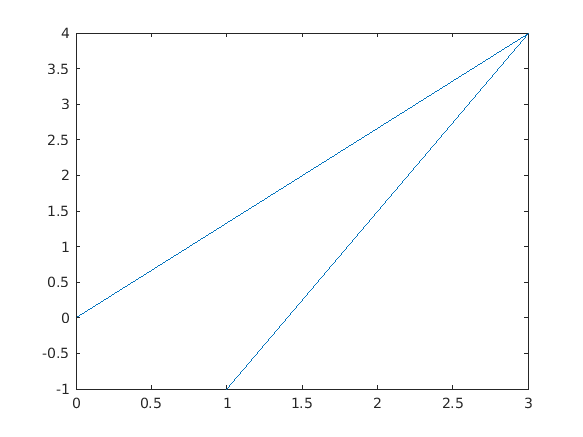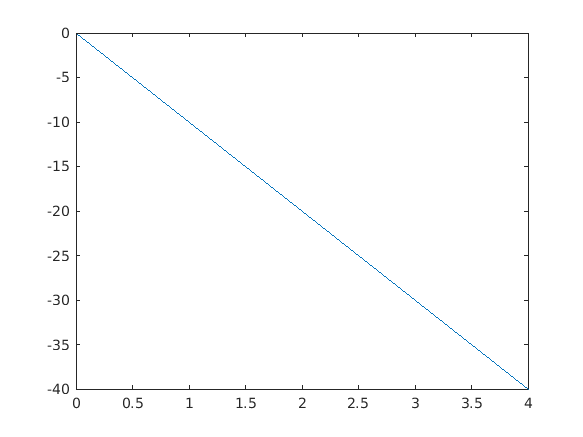Cody

# Problem 769. Calculate the area of a triangle between three points

Solution 2040894

Submitted on 1 Dec 2019 by Vít Lochman
This solution is locked. To view this solution, you need to provide a solution of the same size or smaller.

### Test Suite

Test Status Code Input and Output
1   Pass
X=[1 0 0]; Y=[0 0 1]; y_correct = 0.5; assert(abs(your_fcn_name(X,Y)-y_correct)<1e-3)

x1 = 1 x2 = 0 x3 = 0 y1 = 0 y2 = 0 y3 = 1 V1 = -1 0 0 V2 = -1 1 0 C = 0 0 -1 G = 1 y = 0.5000

``
2   Pass
X=[1 1.5 2]; Y=[0 1 0]; y_correct = 0.5; assert(abs(your_fcn_name(X,Y)-y_correct)<1e-3)

x1 = 1 x2 = 1.5000 x3 = 2 y1 = 0 y2 = 1 y3 = 0 V1 = 0.5000 1.0000 0 V2 = 1 0 0 C = 0 0 -1 G = 1 y = 0.5000

``
3   Pass
X=[2 3 1]; Y=[0 3 4]; y_correct = 3.500; assert(abs(your_fcn_name(X,Y)-y_correct)<1e-3)

x1 = 2 x2 = 3 x3 = 1 y1 = 0 y2 = 3 y3 = 4 V1 = 1 3 0 V2 = -1 4 0 C = 0 0 7 G = 7 y = 3.5000

``
4   Pass
X=[10 12 -10]; Y=[-7 -3 -47]; y_correct = 0; assert(abs(your_fcn_name(X,Y)-y_correct)<1e-3)

x1 = 10 x2 = 12 x3 = -10 y1 = -7 y2 = -3 y3 = -47 V1 = 2 4 0 V2 = -20 -40 0 C = 0 0 0 G = 0 y = 0

``

### Community Treasure Hunt

Find the treasures in MATLAB Central and discover how the community can help you!

Start Hunting!